## Example Questions

### Example Question #1 : How To Find The Perimeter Of A Square

What is the perimeter of a square that has an area of 81?

40.5

36

9

18

36

Explanation:

36

A square has four equal sides and its area = side2. Therefore, you can find the side length by taking the square root of the area √81 = 9. Then, find the perimeter by multiplying the side length by 4:

4 * 9 = 36

### Example Question #2 : How To Find The Perimeter Of A Square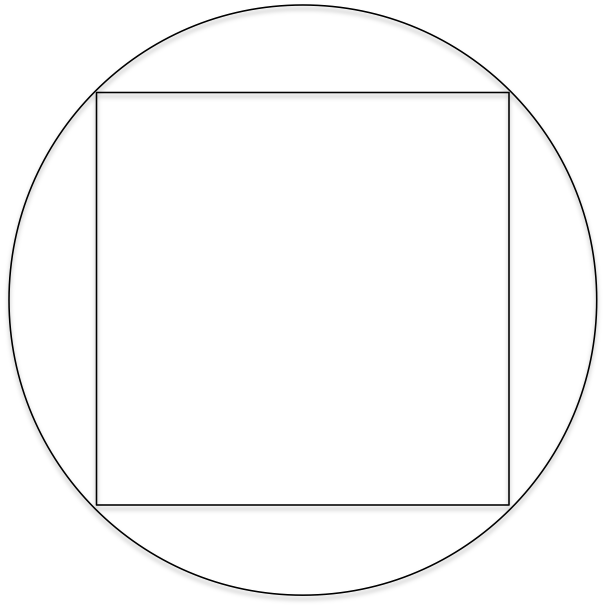The radius of the circle is 2 inches. What is the perimeter of the inscribed square?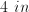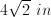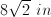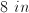Explanation:

The center of an inscribed square lies on the center of the circle. Thus, the line joining the center to a vertex of the square is also the radius. If we join the center with two adjacent vertices we can create a 45-45-90 right isosceles triangle, where the diagonal of the square is the hypotenuse. Since the radius is 2, the hypotenuse (a side of the square) must be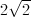.

Finally, the perimeter of a square is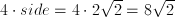### Example Question #3 : How To Find The Perimeter Of A Square

The diagram above represents a square ABCD with a semi-circle directly attached to its side.  If the area of the figure is 16 + 2π, what is its outer perimeter?

12 + 2π

16

20π

16 + 2π

12 + 2π

Explanation:

We know that our area can be represented by the following equation: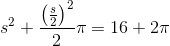Instead of solving the algebra, you should immediately note several things.  16 = 42 and 4 = 22. If the side of the square is 4, then s = 4 would work out as: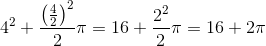which is just what we need.

With s = 4, we know that 3 sides of our figure will have a perimeter of 12.  The remaining semicircle will be one half of the circumference of a circle with diameter of 4; therefore it will be 0.5 * 4 * π or 2π.

Therefore, the outer perimeter of our figure is 12 + 2π.

### Example Question #171 : Plane Geometry

A square table has an area ofsquare centimeters and a perimeter ofcentimeters.

If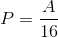, what is the perimeter of the square?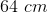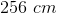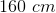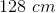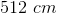Explanation:

We start by writing the equations for the area and perimeter in terms of a side of length s.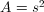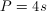Then, substitute both of these expressions into the given equation to solve for side length.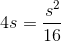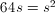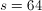Finally, since four sides make up the perimeter, we substitue s back into our perimeter equation and solve for P.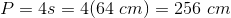### Example Question #5 : How To Find The Perimeter Of A Square

The diagonal of squareis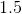feet. Approximately how long in inches is the perimeter of square?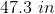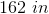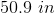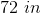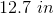Explanation:

First we must convert to inches.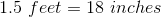The diagonal of a square divides the square into two isosceles-right triangles. Using the Pythagorean Theorem, we know that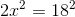, where x is equal to the length of one side of the square.

This gives us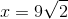.

Therefore, the perimeter of squareis equal to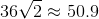.

Tired of practice problems?

Try live online GRE prep today.